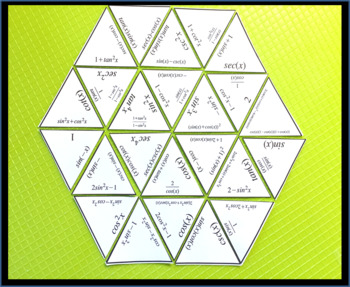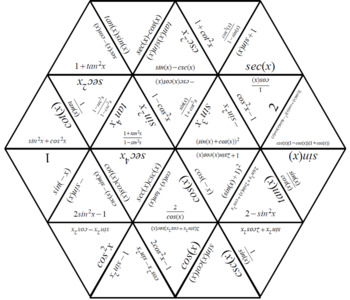# Trigonometric Identities Activity

Rated 4.82 out of 5, based on 10 reviews
10 Ratings;
10th - 12th, Homeschool
Subjects
Resource Type
Formats Included
• Zip
Pages
4 pages
Report this resource to TPT

### Description

Trig Identities Puzzle Activity -

This activity is designed for students to practice recognizing and simplifying trigonometric identities.

Students cut out the shapes in the printout and put them together by matching questions and answers on corresponding sides to create the shape in the given solution. There are 21 questions to match with their corresponding answers on the puzzle pieces.

Solution Included.

Follow me here on TPT where I have many similar activities!

If you would like to see an example of my bingo! games I have some free sample versions:

- Rational (fractional) and negative exponents here: *** FREE Rational and negative exponents Bingo! game ***

A few of my other resources you may like:

Calculus - Differentiating simple trig functions

Calculus - Differentiation Bingo Activity

Calculus: Basic Integration bingo game activity

Logarithm Bingo Activity

Algebraic Fractions Lesson Sequence

Histograms of Variable Width Lesson

Systems of Equations Puzzle Activity

Algebraic Fractions Puzzle Activity

Roots and Radicals (surds) Puzzle Activity

Volume and Surface Area of a Sphere Activity

Multiplying Binomials by expanding brackets Bingo!

Ten Tors Math

Total Pages
4 pages